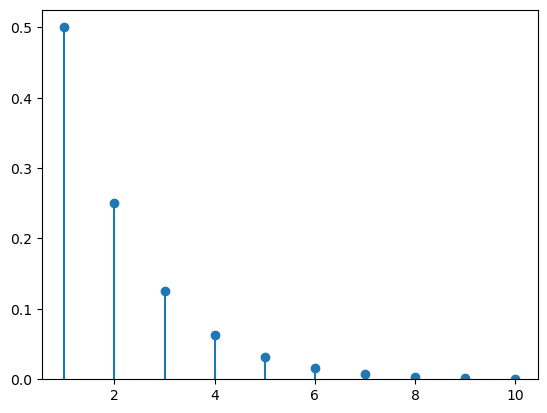# How to plot discrete probability distributions

## Description

There are many famous discrete probability distributions, such as the binomial and geometric distributions. How can we get access to them in software, to plot the distribution as a series of points?

## Solution, in Julia

View this solution alone.

You can import many different random variables from Julia’s Distributions package. The full list of them is online here.

If you don’t have that package installed, first run using Pkg and then Pkg.add( "Distributions" ) from within Julia.

The challenge with plotting a random variable is knowing the appropriate sample space, because some random variables have sample spaces of infinite width, which cannot be plotted.

The example below uses a geometric distribution, whose sample space is $\{1,2,3,\ldots\}$. We specify that we just want to use $x$ values in the set ${1,2,\ldots,10}$. (In some software, the geometric distribution’s sample space begins at 0, but not in SciPy.)

We style the plot below so that it is clear the sample space is discrete.

1
2
3
4
5
6
using Distributions
X = Geometric( 0.5 )      # use a geometric distribution with p=0.5
xs = 1:10                 # specify the range to be 1,2,3,...,10

using Plots
bar( xs, pdf.( X, xs ) )  # plot the shape of the distribution


See a problem? Tell us or edit the source.

## Using SciPy, in Python

View this solution alone.

You can import many different random variables from SciPy’s stats module. The full list of them is online here.

The challenge with plotting a random variable is knowing the appropriate sample space, because some random variables have sample spaces of infinite width, which cannot be plotted.

The example below uses a geometric distribution, whose sample space is $\{1,2,3,\ldots\}$. We specify that we just want to use $x$ values in the set ${1,2,\ldots,10}$. (In some software, the geometric distribution’s sample space begins at 0, but not in SciPy.)

We style the plot below so that it is clear the sample space is discrete.

1
2
3
4
5
6
7
8
9
10
11
12
from scipy import stats
X = stats.geom( 0.5 )     # use a geometric distribution with p=0.5

import numpy as np
xs = np.arange( 1, 11 )   # specify the range to be 1,2,3,...,10

import matplotlib.pyplot as plt
ys = X.pmf( xs )          # compute the shape of the distribution
plt.plot( xs, ys, 'o' )   # plot circles...
plt.vlines( xs, 0, ys )   # ...and lines
plt.ylim( bottom=0 )      # ensure sensible bottom border
plt.show()See a problem? Tell us or edit the source.

## Solution, in R

View this solution alone.

Because R is designed for use in statistics, it comes with many probability distributions built in. A list of them is online here.

The challenge with plotting a random variable is knowing the appropriate sample space, because some random variables have sample spaces of infinite width, which cannot be plotted.

The example below uses a geometric distribution (with $p=0.5$), whose sample space is ${0,1,2,3,\ldots}$. We specify that we just want to use $x$ values in the set ${0,1,2,\ldots,10}$. (In some software, the geometric distribution’s sample space begins at 1, but not in R.)

If you wanted to use a different distribution, you could replace dgeom with, for example, dbinom, adjusting the named parameters as appropriate.

We style the plot below so that it is clear the sample space is discrete.

1
2
3
4
5
xs = 0:8                     # choose the sample space (here, it's 0,1,2,...,10)
ys = dgeom( xs, prob=0.5 )   # compute the shape of the distribution
plot( xs, ys, type='p',      # plot circles...
xlab='sample space', ylab='probability' )
segments( xs, 0, xs, ys )    # ...and lines